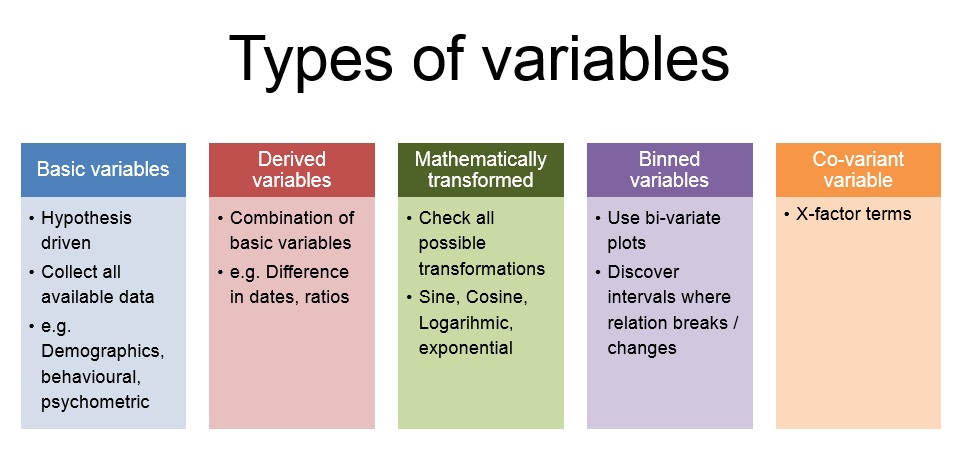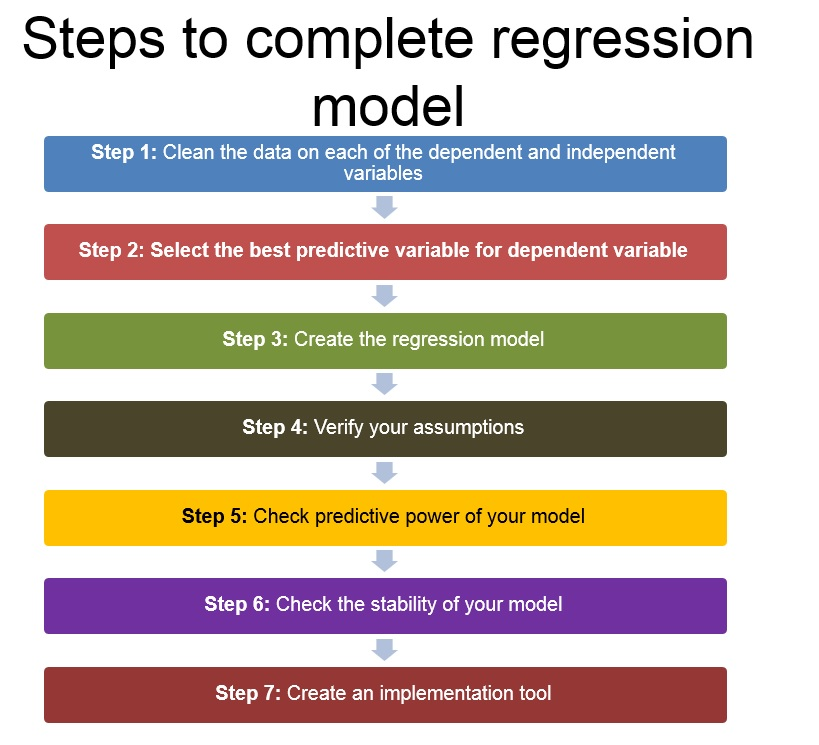Home » Extracting right variables for your Regression model

# Extracting right variables for your Regression model

Getting the right variables in your model and cleaning them can make or break your model.

The precision of the model depends on the breadth (diversity) and depth (spread of data and correct transformations) of variables. This article will take you through some of the techniques used in the industry to create or transform variables. We will also cover the techniques used in the industry to select the right set of variables out of an exhaustive list created in our next article on the subject.Types of variables:

Let’s categorize the possible variables to make the discussion easier and our analysis more structured. Following are the categories of variables we will discuss in this article :

1. Basic variable set

2. Derived variable set

3. Mathematically transformed variable set

4. Bin Variable set

5. Co-variant variable setFollowing is a business case we will consider for creating these variable sets :

Predict the total business of a insurance branch in next 3 months.

1. Creating the basic variable set :

Get this step right and you are half done. There is no set procedure to get the right set of base variables. There are two approaches to get the set of exhaustive base variable set. Following are the approaches :

1. Create hypothesis which can possibly affect the  dependent variable (Here we don’t even care if the data for this variable is even available)

2. Enlist all variables available (Here we don’t even care if this variable can possibly affect the dependent variable)

Here, we try to find all possible variable which can be collected in the analysis without thinking of shortlisting them for the final model. Each of these hypothesis falls in one of the following 3 categories :

a. Demographic variables : These variable defines quantifiable statistics for a data-point.  In the current business case we will include variables like : Location of the branch, Number of Sales managers, Mix of designation in the branch etc.

b. Behavioral variables :  These variables comes from the past performance  of the subject. In the current case we will include variables like business done by branch in last quarter, Ticket size of the business done by branch, performance metrics of the sales managers in the branch.

c. Psychometric variables : For the current business case we will want to include variables like Net Promoter score of the branch, Employee satisfaction score of the branch etc. These types of variables which generally come from surveys are the psychometric variables.

2. Creating the derived variable set :

After completing the list of the basic variables we  move on to the derived variables. These variables have a better predictive power and are very stable. These variables are combination of more than one basic variable. Let’s see how can we form derived variables for the case in hand. Following are the possible variables :

a .Revenue generated per resource = Revenue of the branch / Number of sales managers

b. Revenue on investment of the branch = Revenue of the branch / Total Cost of branch

c. Vintage of the branch = Today’s date  – Date of branch opening

d. Ratio of Senior to Junior employee = # Senior employee/ # Junior employee

This list can go on. Try hard to create all possible combination which are expected to influence the dependent variable. For some more ideas on derived variables, read this article.

3. Creating the Mathematically transformed set :

Till this step we already have all the basic and derived variables. Now is the time to find the best possible transformation for each of these variables. Try to check all possible mathematical transformation such as Sine, Cosine, Logarithmic, Exponential , Square, Square root etc. Once you have all the transformation for each of the variables, you have to choose the transformation that best mimics the dependent variable. This transformation should come from both business sense and statistical method. Following is what I do to choose the best transformation for each variable :

Make a regression model with only 2 steps (step-wise) and the actual dependent variable. Choose the transformation that enter the model in these 2 steps. Check if the transformation found as the best fit makes business sense and use it in the final model. For the current problem in hand Log(Total Revenue) is possibly a better variable than Total Revenue as the marginal addition on prediction will go down as the total revenue increases.

4. Creating the Bin Variable set :

Creating bin variables is very essential in regression model. Find the bivariate plot between dependent variable and all other independent variables listed till now. Find the intervals where the relationship breaks in the bivariate. For the case in hand, lets say the performance of the branch is best if “Ratio of Senior to Junior employee” is between 0.9 to 1.1 . In such case we will create a bin variable between 0.9 and 1.1. It will look like as follows :

Bin = 1    for    0.9 <Ratio < 1.1

Bin = 0    otherwise

We will include this bin variable in the regression model.

5. Creating the Co-variant variable set :

Here comes the X-factor for our regression model. This step incorporate the best cuts of a CART model and significantly raises the prediction power of the regression model. For more details on this technique, please read this article.

Next steps to complete the regression model :

After we are done with the variable collection, following is the order to complete the regression model :

1. Clean the data on each of the dependent and independent variables.

2. Select the best predictive variable for the dependent variable

3. Create the regression model

4. Check the assumptions of the regression model using diagnostic plots (for more details, read this article)

5. Check the predictive power of the model

6. Check the stability of the model

7. Create the implementation toolEnd notes :

The more exhaustive our starting hypothesis the better is the predictive power of the model.Did you find the article useful? Share with us any other variable sets you incorporate in your model.  Do let us know your thoughts about this article in the box below.

## If you like what you just read & want to continue your analytics learning, subscribe to our emails or like our facebook page.

You can also read this article on our Mobile APP•Rachit says:

Hi Kunal,

I would also request you to explain these steps through a case study which can help us in understanding how we
1. clean data
2. select predictors (challenges of dummy variables in case of categorical variables).
3. Verify assumptions
4. Model Validation

•Kunal Jain says:

Rachit,

Thanks for the suggestion. Would cover this through one of the articles in coming days. Stay tuned!

Thanks,
Kunal

•rohit says:

HI Kunal,

Can you just give example of transformation of variables and how do we interpret this transformed variables.

For example:

Y=10 Log(x1)+1000/(x2)+100 sqrt(x3)

And also how should we decide which tranformation goes in favour of our model.

Thanks,
Rohit

•Tavish Srivastava says:

Rohit,
When you have a transformed variable in your kitty, the relationship between x and y is no longer linear. Hence the interpretation of coefficient is made in terms of the transformed variable. In your example, a unit increase in the value of log x1 increases the value of y by 10. Coming to your second question, an easy way to choose transformed variables is to build a step wise regression model for all the transformed variables coming from one input variable and choose the final 2 variables entering the model. This makes sure that the cgi squared value of the transformed variable is high. If the original variable has a much higher chi squared value, the transformed variables will not add much value to your model.

Hope this helps.
Tavish

•Arindam says:

How do you interpret the trignometric function of independent var in business sense. Can you explain with an example.

Thanks.